SPSS TUTORIALS BASICS ANOVA REGRESSION FACTOR CORRELATION

# SPSS Dummy Variable Regression Tutorial

Using categorical predictors in multiple regression requires dummy coding. So how to use such dummy variables and how to interpret the resulting output? This tutorial walks you through.

## Example Data

All examples in this tutorial use staff-dummies.sav, partly shown below.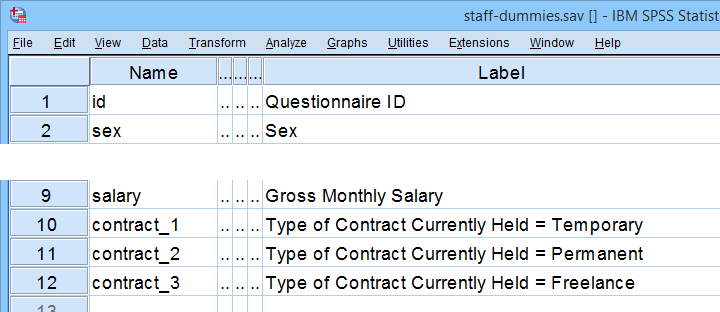Our data file already contains dummy variables for representing Contract Type. Two options for creating such dummy variables in other data files are

## Analysis I - T-Test as Dummy Regression

Let's first examine if monthly salary is related to sex. Two options for finding this out are

These analyses come up with the same results. Comparing these is the first step towards understanding dummy variable regression. Let's first run our t-test from the syntax below.

*Independent samples t-test: salary by sex.

t-test groups sex(1 0)
/variables salary.

## Results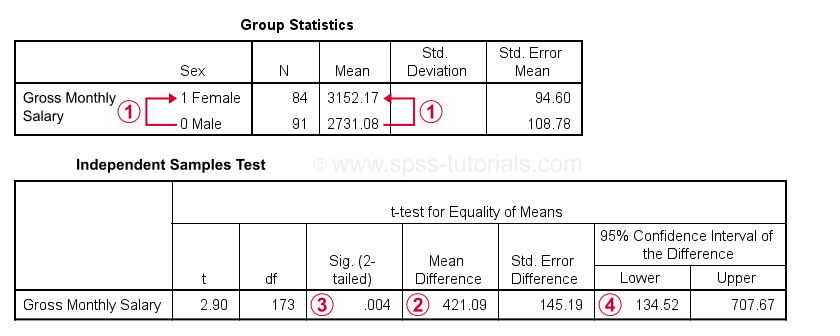Gross monthly salary for females is $421.09 higher than for males. Also note that males are coded 0 while females are coded 1.The significance level for this mean difference is 0.004: we'll probably reject the null hypothesis that the population mean salaries are equal between men and women.A 95% confidence interval suggests a likely range for the population mean difference. It runs from$134.52 through $707.67. Let's now rerun this analysis as regression with a single dummy variable. ## Example I - Single Dummy Predictor In SPSS, we first navigate to AnalyzeRegressionLinear and fill out the dialogs as shown below.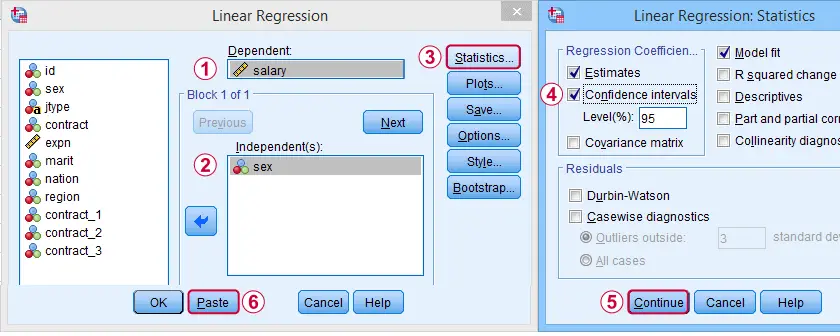Completing these steps results in the syntax below. Let's run it. *Regression: salary by single dummy variable (sex). REGRESSION /MISSING LISTWISE /STATISTICS COEFF OUTS CI(95) R ANOVA /CRITERIA=PIN(.05) POUT(.10) /NOORIGIN /DEPENDENT salary /METHOD=ENTER sex. ## Dummy Variable Regression Output I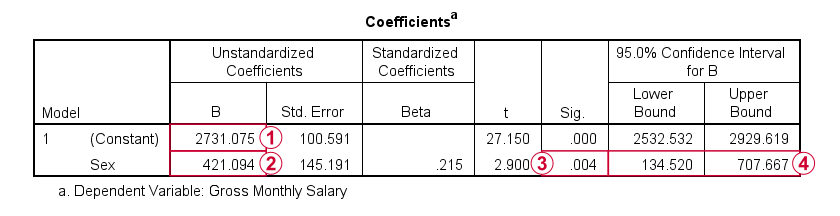Note that the constant is the mean salary for male respondents.The b-coefficient for sex is the mean salary difference between male and female respondents. This is equal to the average increase in salary associated with a 1-unit increase in sex: from male (coded 0) to female (coded 1). This makes sense because the regression equation is $$Salary' = 2731 + 421 \cdot Sex$$ so for all males we predict a gross monthly salary of $$Salary' = 2731 + 421 \cdot 0 = 2731$$ and for all females we predict $$Salary' = 2731 + 421 \cdot 1 = 3152$$ These predicted salaries are simply the mean salaries for male and female respondents. Finally, note thatthe significance level andconfidence interval for the b-coefficient are identical to their counterparts for the mean difference in the t-test results. ## Analysis II - ANOVA as Dummy Regression Let's now see if salary is related to contract type (freelance, temporary or permanent). Precisely, we'll test the null hypothesis that the population mean salaries are equal across all 3 contract types. Two options for testing this hypothesis are: • ANOVA and • dummy variable regression. As we'll see, the b-coefficients obtained from our regression approach are identical to simple contrasts from ANOVA: the mean for a designated reference category is compared to the mean for each other category. These ANOVA results can be replicated from the syntax below. *ANOVA: salary by type of contract. unianova salary by contract /contrast (contract) = simple(1) /print descriptive etasq. ## Results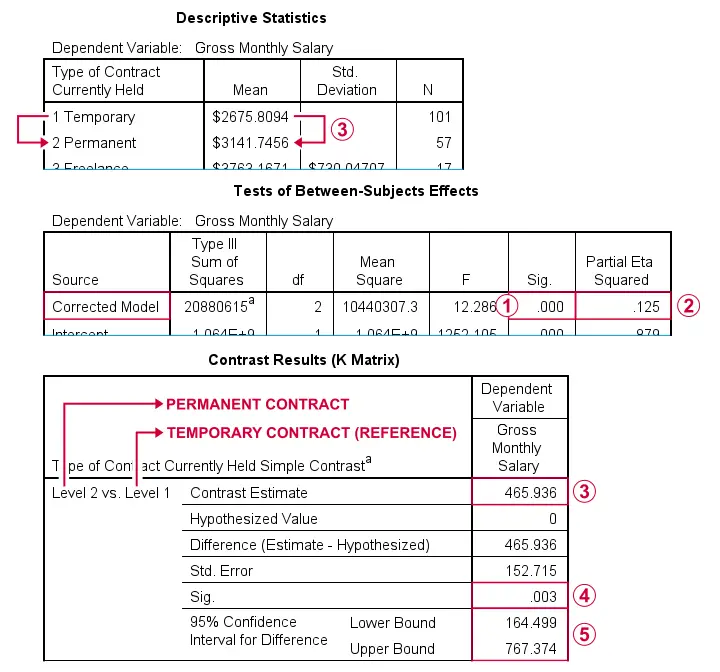Since p < 0.05, we reject the null hypothesis that all population means are equal.The effect size, eta squared is 0.125. This is between medium (0.06) and large (0.14).The mean difference between employees on a permanent versus a temporary contract (the reference category) is$465.94.The p-value and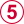confidence interval indicate that this mean difference is “significantly” different from zero, the null hypothesis for this comparison.

In a similar vein, the mean salaries for employees on a freelance versus a temporary contract are compared (not shown here).

## Example II - Multiple Dummy Predictors

We'll navigate to AnalyzeRegressionLinear and fill out the dialogs as shown below.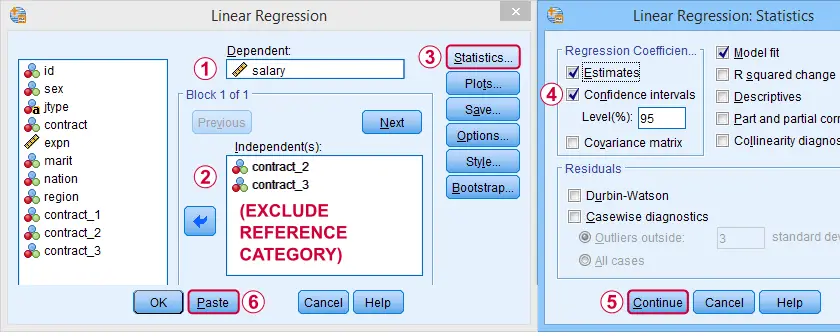We need to choose one reference category and not enter it as a predictor: for representing k categories, we always enter (k - 1) dummy variables.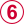Competing these steps generates the syntax below.

*Regression with 2 dummy variables representing type of contract.

REGRESSION
/MISSING LISTWISE
/STATISTICS COEFF OUTS CI(95) R ANOVA
/CRITERIA=PIN(.05) POUT(.10)
/NOORIGIN
/DEPENDENT salary
/METHOD=ENTER contract_2 contract_3.

## Dummy Variable Regression Output II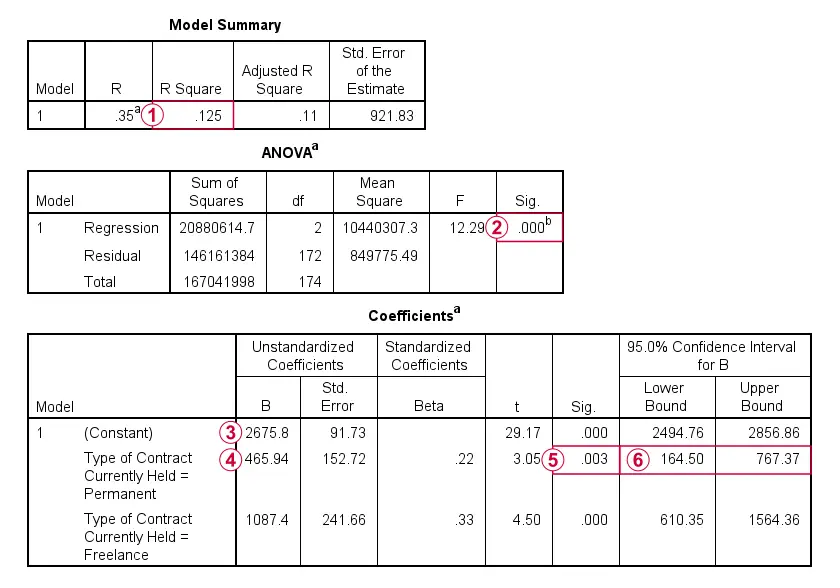Note that r-squared is equal to the ANOVA eta squared we saw earlier. This is always the case: both measures indicate the proportion of variance in the dependent variable accounted for by the independent variable(s).R-squared for the entire model (containing only 2 dummy variables) is statistically significant. In fact, the entire regression ANOVA table is identical to the one obtained from an actual ANOVA.The constant is the mean salary for our reference category: employees having a temporary contract. These respondents score zero on both dummy variables in our model. For them, the regression equation boils down to

$$Salary' = 2675.8 + 465.94 \cdot 0 + 1087.4 \cdot 0 = 2675.8$$The mean salary difference between employees on permanent (dummy) versus temporary (reference) contracts is $321.14 if we control for working experience.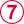Since p < 0.05, this mean difference is statistically significant. Let's now rerun the exact same analysis as an ANCOVA from the syntax below. *ANCOVA for salary by contract, controlling for experience (years). unianova salary by contract with expn /contrast (contract) = simple(1) /print descriptive etasq. ## Results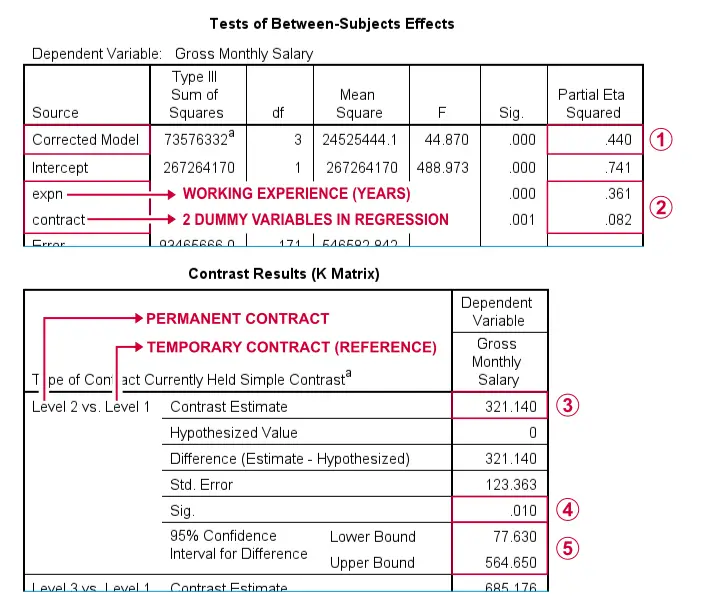Partial eta squared for “corrected model” is equal to the regression r-squared.The output also contains effect sizes for both predictors separately. Note that 0.361 and 0.082 add up to 0.443, somewhat larger than 0.440 for the entire model. This is because these effects partially overlap: experience is associated with contract type.The mean salary difference between employees on permanent versus temporary contracts is$321.14 if we correct for working experience. This difference was seen as a b-coefficient in the previous dummy regression output.Unsurprisingly, the p-value andconfidence interval are identical to their dummy regression counterparts as well.

## Is Dummy Variable Regression Useless?

Many textbooks propose dummy variable regression as the only option for using a combination of quantitative and categorical predictors. However, our last example suggests that ANCOVA may be a better option for this scenario. Why? Well,

• ANCOVA does not require adding (technically redundant) dummy variables to your data.
• ANCOVA comes up with a single effect size (partial eta squared) for the entire categorical predictor. This is more useful than effect sizes for separate dummy variables because we never add them separately to a regression model.
• Testing moderation effects between quantitative and categorical predictors is fairly easy via ANCOVA but rather complicated via regression.

## Final Notes

First off, note that the analyses in this tutorial skipped some important steps:

• we didn't inspect any frequency distributions to see if our data look plausible;
• we did't see if there's any missing values in our data;
• we didn't evaluate any model assumptions (normality, linearity, and so on).

We encourage you to thoroughly examine such issues whenever you're working on real-world data files.

Right, so that should do for dummy regression in SPSS. For a handy overview of the output from all 6 analyses, click here. Did you find this tutorial (not) helpful? Do you agree or disagree with us? Please let us know by throwing a comment below.

# Tell us what you think!

*Required field. Your comment will show up after approval from a moderator.

# THIS TUTORIAL HAS 4 COMMENTS:

•### By YY Ma on April 8th, 2021

A very instructive post!
I have learnt much from it.

•### By DR BODDU NARASIMHULU on April 16th, 2021

Good

•### By MWETEGYEREZE FRANK on May 1st, 2021

It's really interesting

•### By N M on May 18th, 2021

Thank you so much.Been looking high and low to figure out dummy variable regression interpretation. So would you say that in regression its ideally best not to add multiple categorical variables in the same model?